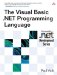# Conversion Module

 <  Day Day Up  >

 The Conversion module contains methods useful for converting values from one type to another. ` Public Function ErrorToString() As String Public Function ErrorToString(ByVal ErrorNumber As Integer) As String ` The ErrorToString function returns a text description of the last exception if no parameter is specified, or a text description of the exception corresponding to the error number. ` Public Function Fix(ByVal Number As Short) As Short Public Function Fix(ByVal Number As Integer) As Integer Public Function Fix(ByVal Number As Long) As Long Public Function Fix(ByVal Number As Decimal) As Decimal Public Function Fix(ByVal Number As Single) As Single Public Function Fix(ByVal Number As Double) As Double Public Function Fix(ByVal Number As Object) As Object ` The Fix function removes the fractional part of a number and returns the whole part of the number. For negative numbers , Fix returns the first negative integer greater than or equal to the number (i.e., “5.5 to “5). ` Public Function Hex(ByVal Number As Byte) As String Public Function Hex(ByVal Number As Short) As String Public Function Hex(ByVal Number As Integer) As String Public Function Hex(ByVal Number As Long) As String Public Function Hex(ByVal Number As Object) As String ` The Hex function converts an integral number into hexadecimal (base 16). ` Public Function Int(ByVal Number As Short) As Short Public Function Int(ByVal Number As Integer) As Integer Public Function Int(ByVal Number As Long) As Long Public Function Int(ByVal Number As Decimal) As Decimal Public Function Int(ByVal Number As Single) As Single Public Function Int(ByVal Number As Double) As Double Public Function Int(ByVal Number As Object) As Object ` The Int function removes the fractional part of a number and returns the whole part of the number. For negative numbers, Int will return the first negative number less than or equal to the number (i.e., “5.5 to “6). ` Public Function Oct(ByVal Number As Byte) As String Public Function Oct(ByVal Number As Short) As String Public Function Oct(ByVal Number As Integer) As String Public Function Oct(ByVal Number As Long) As String Public Function Oct(ByVal Number As Object) As String ` The Oct function converts an integral number into octal (base 8). ` Public Function Str(ByVal Number As Object) As String ` The Str function converts a number or a Boolean value into a string. If the number is positive, a leading space will be added to represent the sign of the number. ` Public Function Val(ByVal Expression As String) As Double Public Function Val(ByVal Expression As Char) As Integer Public Function Val(ByVal Expression As Object) As Double ` The Val function converts a string into a number. The function will read until the first nonwhitespace, nonnumeric value, ignoring all whitespace. It also recognizes hex and octal literals (i.e., &H3A or &O23 ).
 <  Day Day Up  >The Visual Basic .NET Programming Language
ISBN: 0321169514
EAN: 2147483647
Year: 2004
Pages: 173
Authors: Paul Vick

Similar book on Amazon